The bearing capacity of a shallow foundation can be defined as the maximum value of the load applied, for which no point of the subsoil reaches failure point (Frolich method) or else for which failure extends to a considerable volume of soil (Prandtl method and successive).

Prandtl, has studied the problem of failure of an elastic half-space due to a load applied on its surface with reference to steel, characterizing the resistance to failure with a law of the type:

τ=c+σ·tanφ valid for soils as well

Prandtl assumes:

• Weightless material, therefore γ=0;
• rigid – plastic behaviour;
• resistance to failure stated as: τ=c+σ·tanφ;
• uniform vertical load applied to an infinitely long strip of width 2b (Plane strain case);
• no tangential stresses on interface between load strip and bearing surface;
• no overload the edges of the foundation (q’=0).

Upon failure the yield of the material within the space GFBCD is verified. Within the triangle AEB failure occurs according to two families of straight segments inclined by 45°+φ/2 to the horizontal.
Within the triangle AEB failure occurs according to two families of straight segments inclined by 45°+φ/2 to the horizontal.
Within zones ABF and EBC failure occurs along two families of lines, the ones made up of straight lines passing through points A and E, and the other consisting of arcs of families of logarithmic spirals. The poles of these are points A and E. In the triangles AFG and ECD failure occurs along segments inclined at ± (45°+ φ/2 ) to the vertical.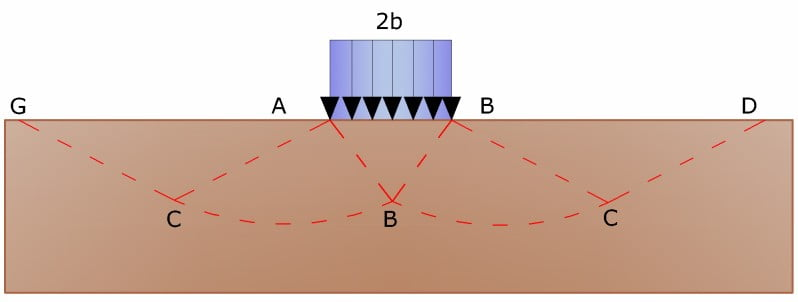Solution of Prandtl

Having thus identified the soil tending to failure by application of the ultimate load, this can be calculated expressing the equilibrium between the forces acting in any volume of soil whose base is delimited by whichever slip surface.
Thus one reaches the equation q =b·c, where the coefficient B depends only upon the soil’s angle of friction φ.

b=cotgφ·[e^(π·tanφ)·tan^(2)(45+φ/2)-1]

For φ =0 coefficient b is 5.14, and therefore q=5.14 · c.

In the alternate case, namely that where the soil is cohesionless (c=0, φ≠0)  q=0, so that according to Prandtl it would not be possible to apply any load to cohesionless soils.
Based on this theory, admittedly of little practical value, all the various investigations and developments have proceeded.
Caquot proceeds from the same premises as Prandtl excepting that the load strip is no longer placed on the surface but at a depth of h ≤ 2b;  the soil between surface and depth h has the following characteristics: γ≠0, φ=0, c=0 i.e. that it is material with weight attribute but no resistance.

The equilibrium equations thus resolve to:

q=A·γ1+b·c

which is certainly a step forward but hardly reflects reality.

Terzaghi (1955), continues on the same lines as Caquot but adds modifications to take into account of the real characteristics of the foundation-soil system.
Under the action of the load transmitted by the foundation, the soil at the contact with the foundation tends to move laterally, but is restrained in this by the tangential resistances that develop between the soil and the foundation. This results in a change of the stress state in the ground placed directly below the foundation.
Terzaghi assigns to the sides AB and EB of Prandtl’s wedge, an inclination ψ to the horizontal, assigning to this a value as a function of the mechanical characteristics of the soil at the contact soil-foundation.

Thus γ2 =0 for soil below the foundation is reviewed assuming that the failure surfaces remain unaltered, the expression for ultimate load becomes:

q=A·γ·h+b·c+C·γ·b

in which, is a coefficient that is a function of the angle of friction φ of the soil below the footing and of the angle φ defined above; and b is the half width of the strip..

Further on the basis of experimental data, Terzaghi introduces factors due to the shape of the foundation. Again Terzaghi refines the original hypothesis of Prandtl who considered the behaviour of soil as rigid–plastic.Terzaghi instead assigns such behaviour only to very compact soils.

In these soils the curve loads/settlements is at first linear, followed by a short curved segment (elastic-plastic behavior). Failure is instantaneous and the value of the ultimate load is easily identifiable (general failure). In a very loose soil however the relation loads/settlements has an accentuated curved line even at low levels of load due to a progressive failure of the soil (local failure) and thus the identification of ultimate load is not so clear like for compact soils.. For very loose soils therefore Terzaghi introduces in the previous formula the reduced values for the mechanical properties of the soil:

tanφrid=(2/3)·tanφ e crid=(2/3)·c

Thus Terzaghi’s formula becomes: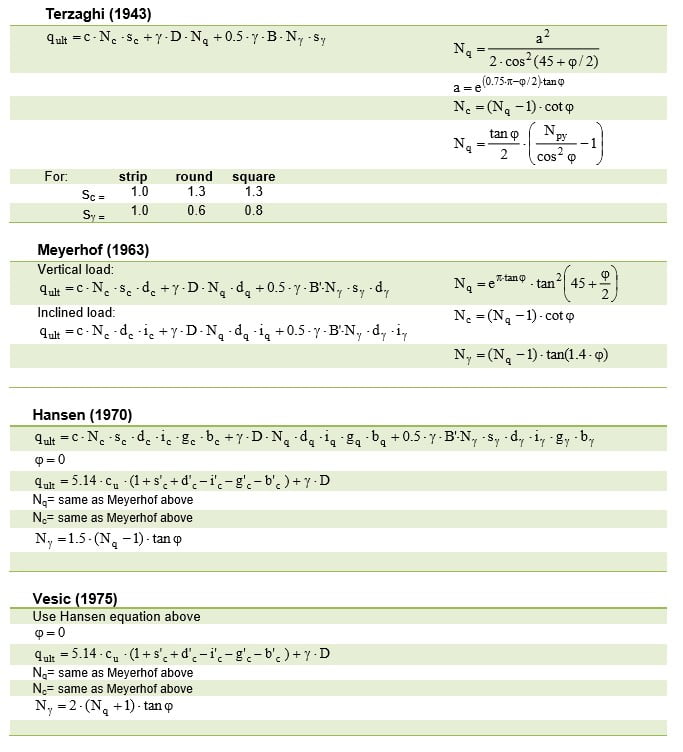Bearing-capacity equations by the several authors indicated

Meyerhof (1963) proposed a formula for calculation of bearing capacity similar to the one proposed by Terzaghi but introducing further foundation shape coefficients.
He introduced a coefficient sq that multiplies the N factor, depth factors  di and inclination factors ii  depth factors di and inclination factors ii for the cases where the load line is inclined to the vertical. Meyerhof obtained the N factors by making trials on a number of BF arcs (see Prandtl mechanism) whilst shear along AF was given approximate values.Shape, depth, and inclination factors for the Meyerhof bearing-capacity

Hansen’s (1970) formula is a further extension on Meyerhof’s; the additions consists in the introduction of bi that accounts for the possible inclination of the footing to the horizontal and a factor gi for inclined soil surface
Hansen’s formula is valid for whatever ratio D/B and therefore for both surface and deep foundations, however the author introduces coefficients to compensate for the otherwise excessive increment in limit load with increased depth.

Vesic (1975) proposes a formula that is analogous to Hansen’s with Nq ed Nc as per Meyerhof and Nγ as below:

Nγ=2·(Nq+1)·tanφ

Shape and depth factors are the same as Hansen’s but there are differences in load inclination, ground inclination and footing inclination factors.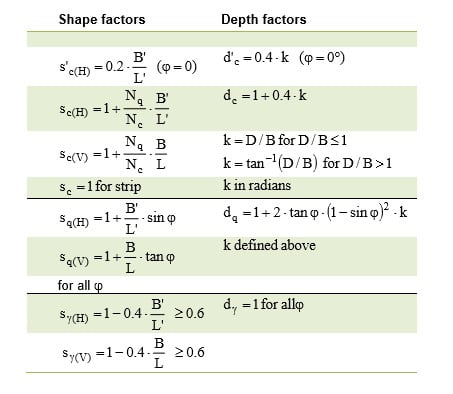Shape and depth factors for use in either the Hansen (1970)
or Vesic (1975) bearing-capacity equations.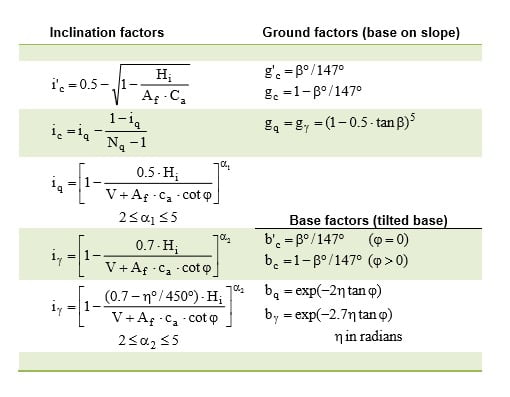Table of inclination, ground, and base factors for the Hansen (1970) equations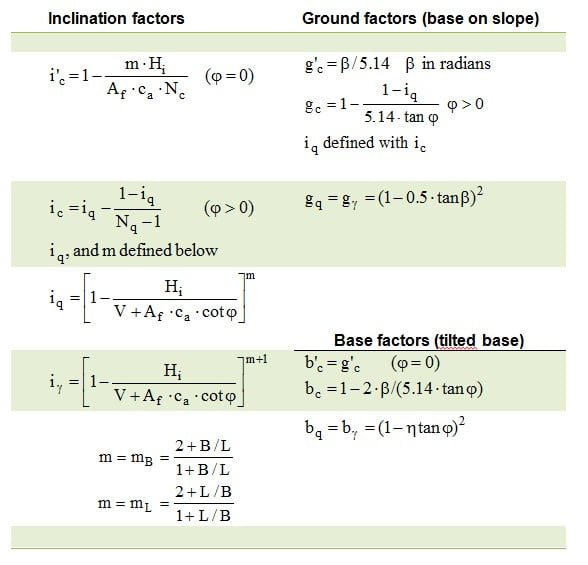Table of inclination, ground, and base factors for the Vesic (1975) equations

Brich-Hansen (EC7-EC8) “In order that a foundation may safely sustain the projected load in regard to general failure for all combinations of load relative to the ultimate limit state, the following must be satisfied:

Vd≤ Rd

Where Vd the design load at ultimate limit state normal to the footing, including the weight of the foundation it self and Rd is the foundation design bearing capacity for normal loads, also taking into account eccentric and inclined loads. When estimating Rd for fine grained soils short and long term situations should be considered.”
Bearing capacity in drained conditions is calculated by:

R/A’=(2+π)·cu·sc·ic+q

Where:

A’ = B’·L’   design effective foundation area. Where eccentric loads are involved, use the reduced area at whose center the load is applied.

cu    undrained cohesion
q     total lithostatic pressure on bearing surface
sc      foundation shape factor
sc     1 + 0,2 (B’/L’) rectangular shapes
sc     1,2 square or circular shapes
ic     correction factor for inclination due to a load H

ic=0.5·[1+(1-H/(A’·cu)^0.5]

Design bearing capacity in drained conditions is calculated as follows:

R/A’ = c’ ·Nc ·sc ·ic + q’· Nq ·sq ·iq + 0,5· g’ ·B’ ·Ng ·sg ·ig

Where:

Nc= same as Meyerhof (1963) above
Nq= same as Meyerhof (1963) above
Nγ=2·(Nq-1)·tanϕ

Shape factors
sq = 1+(B’/L’) ·sinϕ’ rectangular shape
sq = 1+sinϕ’ square or circular shape
sγ =1-0.3·(B’/L’) rectangular shape
sγ =0.7 square or circular shape
sc= (sq ·Nq-1)/(Nq-1) rectangular, square, or circular shape.

In addition to the correction factors reported in the table above will also be considered the ones complementary to the depth of the bearing surface and to the inclination of the bearing surface and ground surface (Hansen).

Sliding considerations
The stability of a foundation should be verified with reference to collapse due to sliding as well as to general failure. For collapse due to sliding, the resistance is calculated as the sum of the adhesion component and the soil-foundation friction component. Lateral resistance arising from passive thrust of the soil can be taken into account using a percentage supplied by the user. Resistance due to friction and adhesion is calculated with the expression:

FRd = Nsd ·tanδ+ca ·A’

In which Nsd is the value of the vertical force, δ is the angle of shearing resistance at the base of the foundation, ca is the foundation-soil adhesion, and A’ is the effective foundation area. There where eccentric loads are involved, use the reduced area at whose centre the load is applied.

Bearing capacity for foundations on rock
Where foundations rest on rock, it is appropriate to take into consideration certain other significant parameters such as the geologic characteristics, type of rock and its quality measured as RQD. It is the practice to use very high values of safety factor for bearing capacity of rock and correlated in some way with the value of RQD (Rock quality designator). For example for a rock whose RQD is up to a maximum of 0.75 the safety factor oscillates between 6 and 10. Terzaghi’s formula can be used in calculation of rock bearing capacity using friction angle and cohesion of the rock or those proposed by Stagg and Zienkiewicz (1968) according to which the coefficients of the bearing capacity are:

Nq=tan^6(45+φ/2)
Nc=5·tan^4(45+φ/2)
Nγ=Nq+1

These coefficients should be used with form factors from the formula of Terzaghi. Ultimate bearing capacity is a function of RQD as follows:

q’=qult(RQD)^2

If rock coring does not render whole pieces (RQD tends to 0) the rock is treated as a soil estimating as best the factors:  c and φ.

Extract of the technical report of  LOADCAP